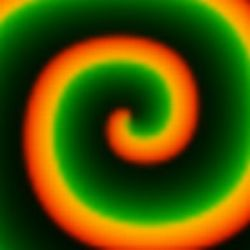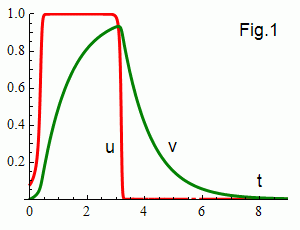framerate

# Barkley's model of an excitable mediumConsider two-variable system of reaction - diffusion equations 
∂u/∂t = (1/ε) u(1 - u)(u - uth ) + Δu ,
∂v/∂t = u - v ,     uth = (v - b)/a .

The local dynamics (in the absence of diffusion) is illustrated in Fig.1. u = v = 0 is the stable fixed point. For u > uth the variable u (the red curve) switches quickly to 1. Due to the small parameter ε it is fast in comparison to the recovery variable v (the green curve) which grows exponentially. When uth = (v + b)/a exceeds u the excitation is quenched to 0 and v decays exponentially. In order to take large time steps a semi-implicit integration scheme is used [1,2].

On 2D plane the u values are displayed by the red color and the v values by the green one. Diffusion propagates excitation through the plane and we get nonlinear waves.

In this script a = 0.5, b = 0.04, ε = 0.02. As since 8 bit floating point values (in RGBA textures) are not enough, therefore floating numbers are stored broken in two cells (bytes).

 Dwight Barkley "A model for fast computer simulation of waves in excitable media" Physica D 49 (1991) 61-70
 M.Dowle, R.M.Mantel and D.Barkley "Fast simulations of waves in three-dimensional excitable media" Int. Journal of Bifurcation and Chaos, Vol. 7, No. 11 (1997) 2529-2545

Simulations on GPU     updated 14 July 2010